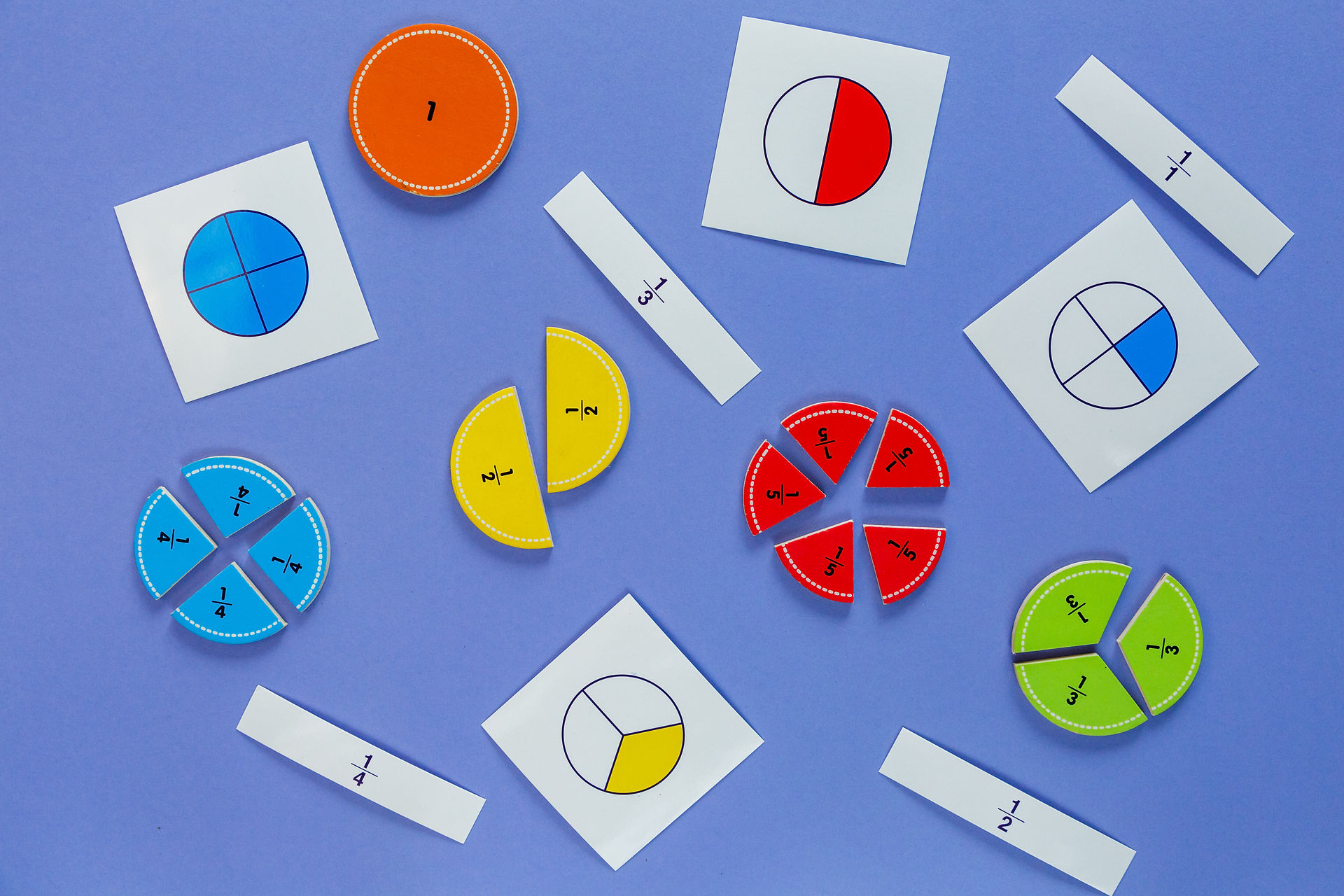### Math Warm-Ups(From Steve Wyborney)Clothesline Math (Estimation 180)# Desmos:  Fractions, Decimals, and Percents on the Number Line# Math Problem of the Day

Number and Operations: Fractions

Common Core Standards

Equivalent Fractions

Fractions on the Number Line, Equivalent Fractions, Partitioning Rectangles

Fraction Concentration

Match fractions to an area or group model.

The Fraction Game

Decomposing fractions, equivalent fractions, combining fractions.

Desmos: The Fraction Challenge

Capture Fractions

Fraction (and Decimal) Models

Connecting fractions, percents, and decimals through visual modeling.

Roll Around the Clock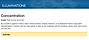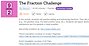Cover Up Fraction Game

Cùbrelo (Spanish)

Explore the relative size of fraction pieces and relationships between them.

Uncover Fraction Game

Descubrelo/Destapalo (Spanish)

Get really good at strategically exchanging fractions for equivalent fractions.

Greatest Fraction Wins!

La Fracción más Grande Gana! (Spanish)

Roll and place each digit as you explore the role of the numerator & denominator  in the value of a fraction as well as model and compare fractions. For 4th and 5th grades, play the Greatest Sum or Greatest Difference wins!

Fraction Capture

Captura la Fracción (Spanish)

This game is like the card game “War.”  Compare fractions, justify your thinking, win cards!

Fractions In-Between Game

Fracciones en el Medio (Spanish)

Compare fractions, order fractions, justify your thinking.

Smallest Product Wins

El Producto Menor Gana!(Fracciones) (Spanish)

Roll the die and place the digit into the template to make the smallest product.  Focuses on multiplying a whole number by a fraction.  You could vary it to multiply a fraction by a fraction.

Fraction War (from Fractiontalks.com)

Fraction War Video

Fraction War Cards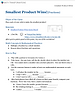Desmos:  Equivalent Fractions

The Kool-Aid Kid

Fraction Estimation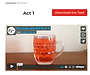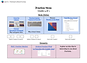Number and Operations in Base Ten: Decimals

Common Core StandardsDesmos:  The Decimal Challenge

Comparing and Ordering Decimal Numbers

Multiplying and Dividing Decimal Numbers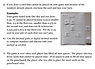Target 1

En el Blanco 1 (Spanish)

Digital Version: Close to 1

Players choose cards to make 2 decimal numbers that have a sum as close to 1 as possible.

Target 2

En el Blanco 2 (Spanish)

The Place Value Game

Juego de Valor Posicional (Spanish)

Place Value Game Demo Video

Virtual Spinner

Decimal Place Value

Comparar con Decimales (Spanish)

Digital Version: Decimal Compare

Digital Tool:  Modeling Decimals

Compare decimals - like the card game War.

Decimal Double Compare

Comparación Decimal Doble (Spanish)

Digital Version: Decimal Double Compare

Decimal Subtraction Compare

Digital Tool:  Modeling Decimals

Smaller to Larger - Decimals

Decimales de Menor a Mayor (Spanish)

Digital Version: Smaller to Larger

Digital Tool:  Modeling Decimals

Decimal Maze

Making sense of decimal operations.  What is the highest score you can get?  Use a calculator and have fun!

Decimals In-between

Other Decimal Games

5th Grade Choice Board: Decimals (with connections to fractions & percents)### Multiplication and Division

Arithmetic (PhET)

Multiplication Table Exploration

Area Model Introduction (PhET)

(PhET)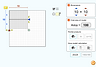### PracticePractice Book (Fractions Units 4 & 7)

Temporarily Free from The Math Learning Center